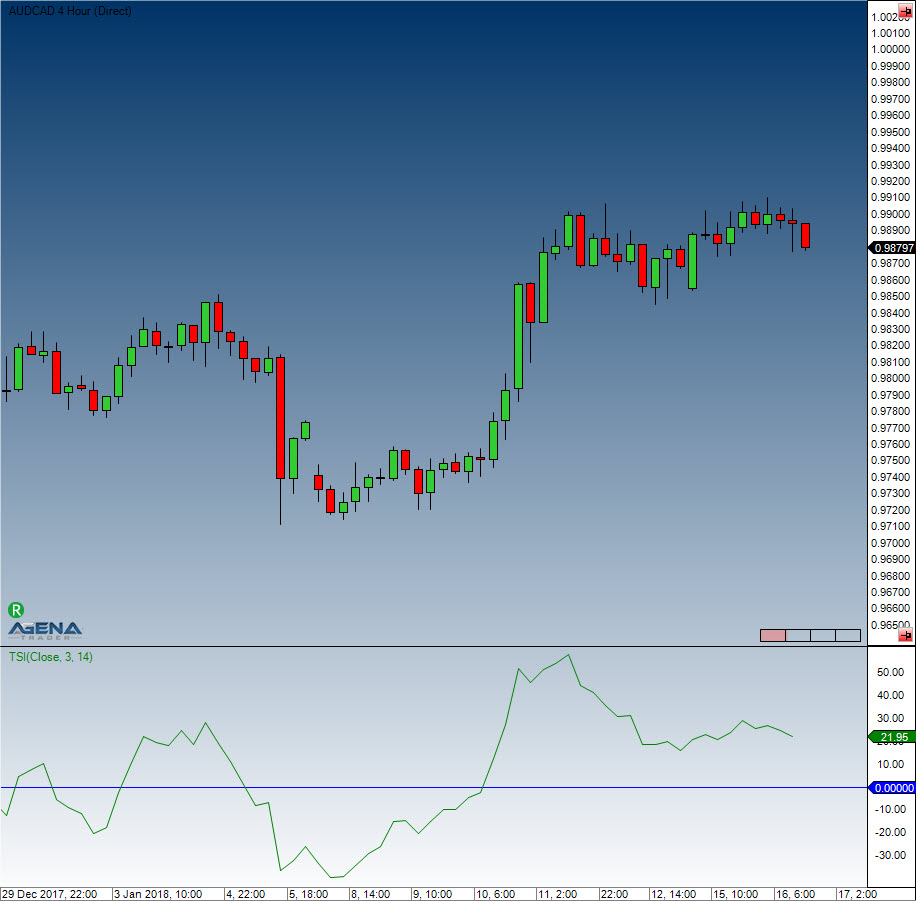# True Strength Index (TSI)

#### Description

The True Strength Index (TSI) is a momentum indicator. It is used as an indicator for trend direction and for displaying overbought or oversold conditions. As a rule, momentum oscillators preemptively indicate price changes, whereas moving averages generally lag behind the price. The TSI brings together the advantages of these two indicator groups.

#### Interpretation

The TSI line moves between 100 and -100. Most values are between +25 and -25. These trigger lines can be used to anticipate overbought or oversold situations. A rising TSI signals an uptrend, while falling TSI suggests a downtrend.

#### Usage

TSI(int fast, int slow)
TSI(IDataSeries inSeries, int fast, int slow)
TSI(int fast, int slow)[int barsAgo]
TSI(IDataSeries inSeries, int fast, int slow)[int barsAgo]

#### Return value

double
When using this method with an index (e.g. TSI(3,14)[int barsAgo] ), the value of the indicator will be issued for the referenced bar.

#### Parameters

inSeries Input data series for the indicator
fast Number of bars included in the calculation of the fast EMA
slow Number of bars included in the calculation of the slow EMA

#### VisualizationTrue Strength Index (TSI)

#### Example

//Output of the current value for the True Strength Index (TSI)
Print("The current value for the TSI is " + TSI(3, 14));